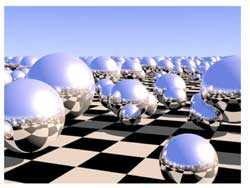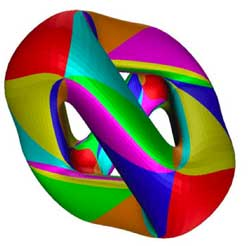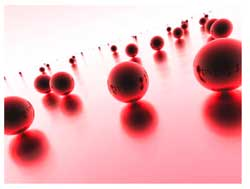The
natural habitat of this lesson is a bit further down the course, but it
was called into existence by a comment/question by
Kea

I don’t yet quite see where the nc
manifolds are, but I guess that’s coming.

As
I’m enjoying telling about all sorts of sources of finite dimensional
representations of $SL_2(\mathbb{Z})$ (and will carry on doing so for
some time), more people may begin to wonder where I’m heading. For this
reason I’ll do a couple of very elementary posts on simple examples of
noncommutative manifolds.

I realize it is ‘bon ton’ these days
to say that noncommutative manifolds are virtual objects associated to
noncommutative algebras and that the calculation of certain invariants
of these algebras gives insight into the topology and/or geometry of
these non-existent spaces. My own attitude to noncommutative geometry is
different : to me, noncommutative manifolds are genuine sets of points
equipped with a topology and other structures which I can use as a
mnemotechnic device to solve the problem of interest to me which is the
classification of all finite dimensional representations of a smooth
noncommutative algebra.

Hence, when I speak of the
‘noncommutative manifold of $SL_2(\mathbb{Z})$’ Im after an object
containing enough information to allow me (at least in principle) to
classify the isomorphism classes of all finite dimensional
$SL_2(\mathbb{Z})$-representations. The whole point of this course is
to show that such an object exists and that we can make explicit
an elementary question :

Riemann surfaces are examples of
noncommutative manifolds, so what is the noncommutative picture of
them?I’ve browsed the Google-pictures a bit and a picture
coming close to my mental image of the noncommutative manifold of a
Riemann surface locally looks like the picture on the left. Here, the checkerboard-surface is part of the Riemann surface
and the extra structure consists in putting in each point of the Riemann
surface a sphere, reflecting the local structure of the Riemann surface
near the point. In fact, my picture is slightly different : I want to
draw a loop in each point of the Riemann surface, but Ill explain why
the two pictures are equivalent and why they present a solution to the
problem of classifying all finite dimensional representations of the
Riemann surface. After all why do we draw and study Riemann
surfaces? Because we are interested in the solutions to equations. For
example, the points of the _Kleinian quartic Riemann
surface_give us all solutions tex \in
\mathbb{C}^3 $to the equation$X^3Y+Y^3Z+Z^3X=0 $. If (a,b,c) is such a solution, then so are all scalar multiples$(\lambda a,\lambda
b,\lambda c) $so we may as well assume that the Z$coordinate is equal
to 1 and are then interested in finding the solutions tex \in
\mathbb{C}^2 $to the equation$X^3Y+Y^3+X=0 $which gives us an affine patch of the Kleinian quartic (in fact, these solutions give us all points except for two, corresponding to the _points at infinity_ needed to make the picture compact so that we can hold it in our hand and look at it from all sides. These points at infinity correspond to the trivial solutions (1,0,0) and (0,1,0)). What is the connection between points on this Riemann surface and representations? Well, if (a,b) is a solution to the equation$X^3Y+Y^3+X=0 $, then we have a _one-dimensional representation_ of the affine _coordinate ring_$\mathbb{C}[X,Y]/(X^3Y+Y^3+X) $, that is, an algebra morphism$\mathbb{C}[X,Y]/(X^3Y+Y^3+X) \rightarrow \mathbb{C} $defined by sending X to a and Y to b. Conversely, any such one-dimensonal representation gives us a solution (look at the images of X and Y and these will be the coordinates of a solution). Thus, commutative algebraic geometry of smooth curves (that is Riemann surfaces if you look at the ‘real’ picture) can be seen as the study of one-dimensional representations of their smooth coordinate algebras. In other words, the classical Riemann surface gives us already the classifcation of all one-dimensional representations, so now we are after the ‘other ones’. In noncommtative algebra it is not natural to restrict attention to algebra maps to$\mathbb{C} $, at least we would also like to include algebra maps to$n \times n $matrices$M_n(\mathbb{C}) $. An n-dimensional representation of the coordinate algebra of the Klein quartic is an algebra map$\mathbb{C}[X,Y]/(X^3Y+Y^3+X) \rightarrow M_n(\mathbb{C}) $That is, we want to find all pairs of$n \times n $matrices A and B satisfying the following matrix-identities$A.B=B.A $and$A^3.B+B^3+A=0_n $The first equation tells us that the two matrices must commute (because we took commuting variables X and Y) and the second equation really is a set of$n^2 $-equations in the matrix-entries of A and B. There is a sneaky way to get lots of such matrix-couples from a given solution (A,B), namely by _simultaneous conjugation_. That is, if$C \in GL_n(\mathbb{C}) $is any invertible$n \times n $matrix, then also the matrix-couple$~(C^{-1}.A.C,C^{-1}.B.C) $satisfies all the required equations (write the equations out and notice that middle terms of the form$C.C^{-1} $cancel out and check that one then obtains the matrix-identities$C^{-1} A B C = C^{-1} BA C $and$C^{-1}(A^3B+B^3+A)C = 0_n $which are satisfied because (A,B) was supposed to be a solution). We then say that these two n-dimensional representations are _isomorphic_ and naturally we are only interested in classifying the isomorphism classes of all representations. Using classical commutative algebra theory of Dedekind domains (such as the coordinate ring$\mathbb{C}[X,Y]/(X^3Y+Y^3+X) $) allows us to give a complete solution to this problem. It says that any n-dimensional representation is determined up to isomorphism by the following geometric/combinatorial data • a finite set of points$P_1,P_2,\dots,P_k $on the Riemann surface with$k \leq n $. • a set of positive integers$a_1,a_2,\dots,a_k $associated to these pointssatisfying$a_1+a_2+\dots_a_k=n $. • for each$a_i $a partition of$a_i $(that is, a decreasing sequence of numbers with total sum$a_i $). To encode this classification I’ll use the mental picture of associating to every point of the Klein quartic a small loop.$\xymatrix{\vtx{}
\ar@(ul,ur)} $Don\’t get over-exited about this noncommutative manifold picture of the Klein quartic, I do not mean to represent something like closed strings emanating from all points of the Riemann surface or any other fanshi-wanshi interpretation. Just as Feynman-diagrams allow the initiated to calculate probabilities of certain interactions, the noncommutative manifold allows the initiated to classify finite dimensional representations. Our mental picture of the noncommutative manifold of the Klein quartic, that is : the points of the Klein quartic together with a loop in each point, will tell the initiated quite a few things, such as : The fact that there are no arrows between distict points, tells us that the classification problem splits into local problems in a finite number of points. Technically, this encodes the fact that there are no nontrivial extensions between different simples in the commutative case. This will drastically change if we enter the noncommutative world…The fact that there is one loop in each point, tells us that the local classification problem in that point is the same as that of classifying nilpotent matrices upto conjugation (which, by the Jordan normal form result, are classified by partitions) Moreover, the fact that there is one loop in each point tells us that the local structure of simple representations near that point (that is, the points on the Kleinian quartic lying nearby) are classified as the simple representations of the polynmial algebra$\mathbb{C}[x] $(which are the points on the complex plane, giving the picture of the Riemann sphere in each point reflecting the local neighborhood of the point on the Klein quartic) In general, the noncommutative manifold associated to a noncommutative smooth algebra will be of a similar geometric/combinatorial nature. Typically, it will consist of a geometric collection of points and arrows and loops between these points. This data will then allow us to reduce the classification problem to that of _quiver-representations_ and will allow us to give local descriptions of our noncommutative manifolds. Next time, I’ll give the details in the first noncommutative example : the skew-group algebra of a finite group of automorphisms on a Riemann surface (such as the simple group$PSL_2(\mathbb{F}_7) \$ acting on the
Klein quartic). Already in this case, some new phenomena will
appear…

ADDED : While writing this post
NetNewsWire informed me that over at Noncommutative Geometry they have a
post on a similar topic : What is a noncommutative space.

Published in featured

## One Comment

This site uses Akismet to reduce spam. Learn how your comment data is processed.